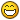## Recommended Posts

I have some doubt about the depth shader. The output seems not to be the depth value in NDC space, nor the value like gl_FragCoord.z. So what it is about? Please correct me if I am wrong.``````gl_Position = viewProjection * finalWorld * vec4(position, 1.0);

vDepthMetric = ((gl_Position.z + depthValues.x) / (depthValues.y));``````

##### Share on other sites

Hello!

The value is in linear space

See here an example on how to use it: https://github.com/BabylonJS/Babylon.js/blob/master/src/Shaders/ssao2.fragment.fx#L43

##### Share on other sites

5 hours ago, Deltakosh said:

Hello!

The value is in linear space

See here an example on how to use it: https://github.com/BabylonJS/Babylon.js/blob/master/src/Shaders/ssao2.fragment.fx#L43

Thank you @Deltakosh. Hummm...I am really confused. As far as I understand,  the z component in projection space (some sort of 1/Zviewspace) is linear in screen space (for interpolation reason) but non linear as depth. So this is for convert it again to a linear depth but mapped in [0, 1]?

The value of vDepthMetric is

1 - ((far - gl_Position.z) / (far + near)). I am not sure why it is linear again. Maybe it is a stupid question though...Thanks in advance for more explanation.One more question, is gl_FragCoord.z the interpolation result of z in projection space?

##### Share on other sites

8 hours ago, Deltakosh said:

Hello!

The value is in linear space

See here an example on how to use it: https://github.com/BabylonJS/Babylon.js/blob/master/src/Shaders/ssao2.fragment.fx#L43

Hi @Deltakosh, the sample you gave was about the SSAO2, it uses the GeometryBufferRenderer which is based on the shader geometry.vertex.fx and geometry.fragment.fx.  Please see the links below. Here we output directly depth in view space (although I don't understand why we need to divide z by w because w should always be 1.0 here). It works perfectly with the SSAO2 sample as it construct a viewRay in View space.

My initial question is about depth.vertex.fx and depth.fragment.fx, which I believe are used by DepthRenderer. Then the output of DepthRenderer is used by SSAO effect.

in depth.vertex.fx

``````gl_Position = viewProjection * finalWorld * vec4(position, 1.0);

vDepthMetric = ((gl_Position.z + depthValues.x) / (depthValues.y));``````
``````float depth = texture2D(textureSampler, vUV).r;
vec3 position = vec3(vUV, depth);``````

##### Share on other sites

8 hours ago, Deltakosh said:

Hello!

The value is in linear space

See here an example on how to use it: https://github.com/BabylonJS/Babylon.js/blob/master/src/Shaders/ssao2.fragment.fx#L43

Hi @Deltakosh, the sample you gave was about the SSAO2, it uses the GeometryBufferRenderer which is based on the shader geometry.vertex.fx and geometry.fragment.fx.  Please see the links below. Here we output directly depth in view space. This should be the linear depth. It works with the SSAO2 sample as it construct a viewRay in View space.

My initial question is about depth.vertex.fx and depth.fragment.fx, which I believe are used by DepthRenderer. Then the output of DepthRenderer is used by SSAO effect.

in depth.vertex.fx

``````gl_Position = viewProjection * finalWorld * vec4(position, 1.0);

vDepthMetric = ((gl_Position.z + depthValues.x) / (depthValues.y));``````

in ssao.fragment.fx

``````float depth = texture2D(textureSampler, vUV).r;
vec3 position = vec3(vUV, depth);``````

As we know that vUV is [0, 1], so should be the "depth". My question is why we don't directly use gl_FragCoord.z? Why we use the "vDepthMetric"?

##### Share on other sites

Hello unfortunately gl_FragCoord.z is NOT linear

(z = (clip.z/clip.w)/2 + 0.5)

## Join the conversation

You can post now and register later. If you have an account, sign in now to post with your account.
Note: Your post will require moderator approval before it will be visible.×   Pasted as rich text.   Paste as plain text instead

Only 75 emoji are allowed.